# Texas Go Math Kindergarten Lesson 2.5 Answer Key Understand, Identify, and Write 0

Refer to our Texas Go Math Kindergarten Answer Key Pdf to score good marks in the exams. Test yourself by practicing the problems from Texas Go Math Kindergarten Lesson 2.5 Answer Key Understand, Identify, and Write 0.

## Texas Go Math Kindergarten Lesson 2.5 Answer Key Understand, Identify, and Write 0

Unlock the Problem

DIRECTIONS: Use counters to model this problem. There are two horses in the pen. Then the two horses leave the pen and go to the field. How many horses are in the pen now? Trace and practice writing the number. Trace the word. Tell a friend what you know about that number.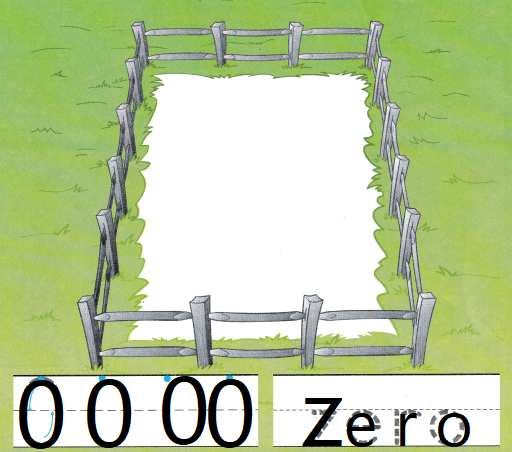Explanation:
Traced the number zero
and number name zero

Try Another Problem

DIRECTIONS: 1. There is one backpack on each hook. Use counters to model the backpacks. Draw the counters. How many backpacks are there? Write the number. 2. There is one backpack on each hook. Use counters to model a backpack on each hook. Three children each take one backpack off a hook. How many backpacks are there now? Write the number.

Question 1.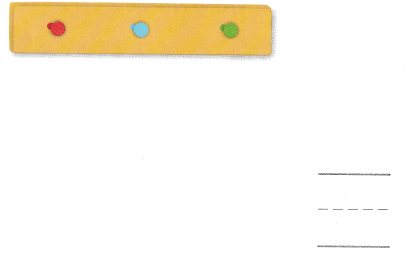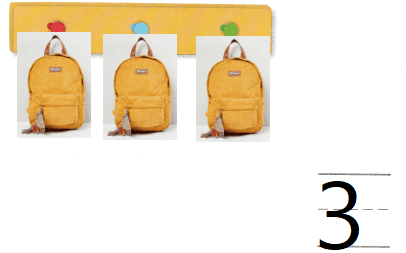Explanation:
There is one backpack on each hook.
Use counters to model the backpacks.
Drawn the counters.
there are 3 backpacks

Question 2.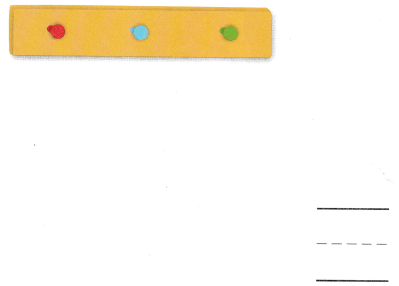Explanation:
There is one backpack on each hook.
Use counters to model a backpack on each hook.
Three children each take one backpack off a hook.
0 backpacks are there now.
Share and Show

DIRECTIONS: Use counters. 3. Drew has one book. Adam has one fewer book than Drew. How many books does Adam have? Write the number. 4. Bradley has no pencils. Matt has one more pencil than Bradley. How many pencils does Matt have? Write the number.

Question 3.Explanation:
Drew has one book.
Adam has one fewer book than Drew.

Question 4.Explanation:
Matt has one more pencil than Bradley.
1 pencils Matt have

HOME ACTIVITY • Have your child place up to five macaroni pieces in a cup. Remove some or oil of the macaroni and have him or her tell how many pieces are in the cup and write the number.
5 – 1 = 4
Explanation:
There are 4 macaroni in the cup

DIRECTIONS: Choose the correct answers. 5. How many balls are in the basket? 6. Which basket shows a items? 7. Jake has no pets. How many pets does Jake have?

Question 5.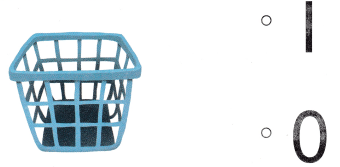Explanation:
bubbled the option where the 0 is shown

Question 6.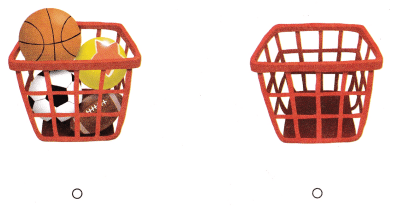Explanation:
bubbled the option where the 4 is shown

Question 7.Explanation:
Jake has no pets.
Jake have 0 pets

### Texas Go Math Kindergarten Lesson 2.5 Homework and Practice Answer Key

DIRECTIONS: Use counters. 1. The brown nest has no eggs. The green nest has two more eggs than the brown nest. How many eggs are in the green bird nest? Write the number. 2. Tim has five balloons. All of the balloons fly away. How many balloons does Tim have now? Write the number.

Question 1.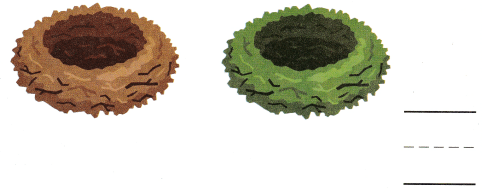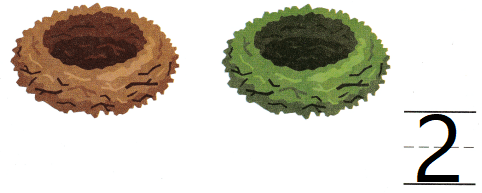Explanation:
The brown nest has no eggs.
The green nest has two more eggs than the brown nest.
2  eggs are in the green bird nest

Question 2.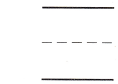Explanation:
Tim has five balloons.
All of the balloons fly away.
0 many balloons does Tim have now

DIRECTIONS: Choose the correct answer. 3. How many shoes are in the box? 4. Which tray shows 0 items? 5. Diana has no pencils. How many pencils does Diana have?

Lesson Check

Question 3.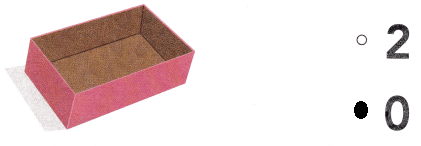Explanation:
There are 0 shoes in the shop

Question 4.Explanation:
The first tray shows the 0 items

Question 5.